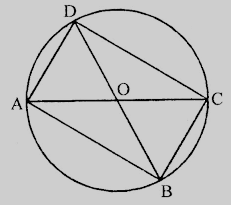# If the two sides of a pair of opposite sides of a cyclic quadrilateral are equal, prove that is diagonals are equal.

Given:

The two sides of a pair of opposite sides of a cyclic quadrilateral are equal.

To do:

We have to prove that is diagonals are equal.

Solution:

Let in a cyclic quadrilateral $ABCD, AB = CD, AC$ and $BD$ are the diagonals.$AB = CD$

arc $AB =$ arc $CD$

Adding arc $BC$ to both sides, we get,

arc $AB +$ arc $BC =$ arc $BC +$ arc $CD$

arc $AC =$ arc $BD$

Therefore,

$AC = BD$

Hence the diagonals of the cyclic quadrilateral are equal.

Updated on: 10-Oct-2022

31 Views